### Upcoming midterm exam

when and where The first midterm exam (out of two) is coming up Monday March 2. You will have the whole 50 minute class period to take the exam. The exam will be in our regular classroom.

what The exam will cover:

• All material in Chapters 1-3 of the Gotelli book including basic matrix population modeling concepts.
• All material covered in lectures including today’s lecture on matrix population models.
• All material covered in labs 1-3.
• Ch. 1 of “Beyond Connecting the Dots” (basic systems thinking and stock-flow modeling, concepts of feedbacks and equilibria)
• Basic programming concepts (IF-THEN-ELSE and iteration loops)

The exam will consist of a mixture of multiple-choice and short-answer questions.

Please bring a calculator- a regular scientific calculator is fine (not absolutely necessary, but it could be helpful for at least one question on the exam!)

Any questions?

# Matrix population models

First of all, this lecture is full of R code (R is pretty good at running matrix population models!). If you want to follow along in R, you can find the R script here. I recommend right-clicking on the link, saving the script to a designated folder, and loading up the script in RStudio.

## Why matrices?

### Reason 1: simplify!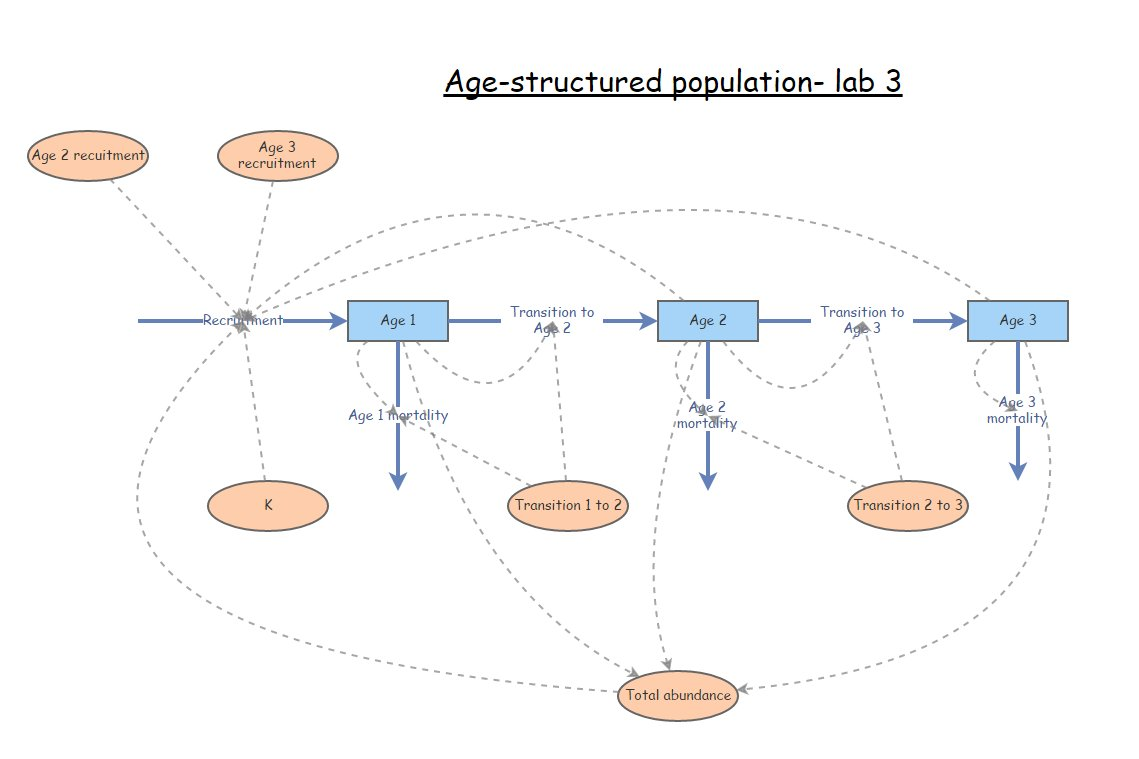You might recognize this InsightMaker model from Lab 3. This represents an age-structured population with only three age classes. Imagine if there were five age classes, or 10? What if you could jump from (e.g.) stage 3 to stage 5 or 5? How many lines would you have to draw, how many equations would you have to put in all the different flows? It would be tedious, and you could easily run into errors that would be very hard to debug!Consider the teasel example from our textbook. It’s possible to implement this model in InsightMaker, but how tedious would this be? And this is far from the most complicated populations out there (although notice that plants can do some things that animals can’t do- for instance go backwards in developmental stage. With matrix models, there is an easier way!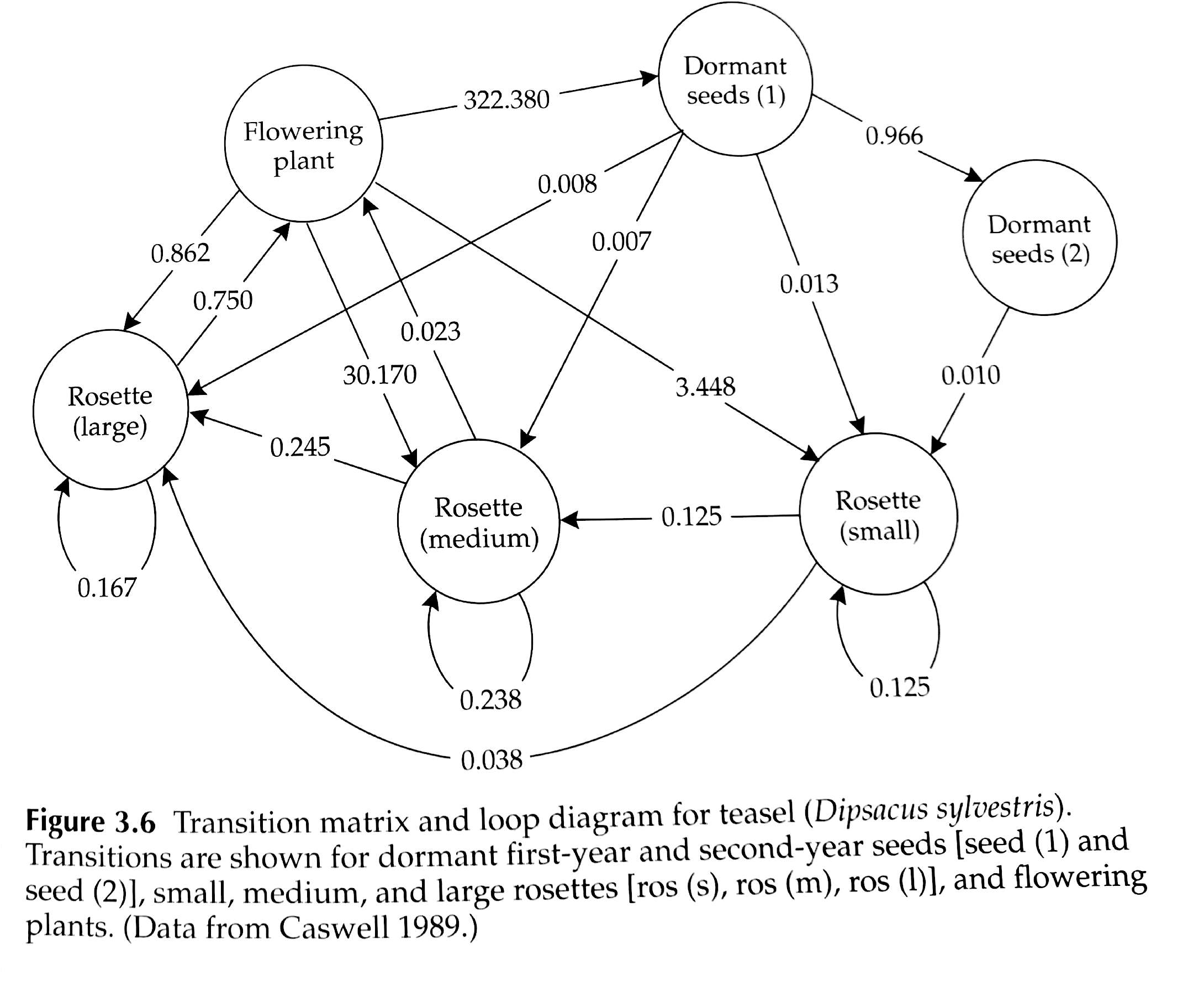The population vital rates for pretty much any age-structured or stage-structured population can be represented as a transition matrix (or, projection matrix), which summarizes all the information about mortality, birth rates, and transitions between stages! (and the fact that a life history like teasel can be represented by a transition matrix illustrates the generality of this concept!)

For example, the teasel vital rates can be summarized in this matrix:

#########
# Teasel example from Gotelli: summarizing a complex life history!

teasel <- teasel[,-1]                                  # remove the row names
teasel_matrix <- as.matrix(teasel)                     # convert to a matrix (from a data frame)
colnames(teasel_matrix) <- names(teasel)               # assign row and column names
rownames(teasel_matrix) <- names(teasel)
teasel_matrix                                          # print the matrix
##           Seed1 seed2  ros1  ros2  ros3 flowering
## Seed1     0.000  0.00 0.000 0.000 0.000   322.380
## seed2     0.966  0.00 0.000 0.000 0.000     0.000
## ros1      0.013  0.01 0.125 0.000 0.000     3.448
## ros2      0.007  0.00 0.125 0.238 0.000    30.170
## ros3      0.008  0.00 0.000 0.245 0.167     0.862
## flowering 0.000  0.00 0.000 0.023 0.750     0.000

Isn’t that elegant!!

We’ll go into more detail about matrices later!

### Reason 2: projection!

In one of the questions in Lab 3, your were asked to use a life table to project the age structure of a population one time step in the future. Was it simple and straightforward to do this? (answer: NO!!)

Life tables are great for summarizing survivorship schedules and other aspects of age-structured populations. But life tables are not great for projecting age-structured abundance into the future!

You know what is great for projecting age-structured abundance into the future? (obvious answer: MATRICES!)

For example, let’s project a teasel population 1 year into the future:

First of all, we need to begin with a teasel population vector

#############
# Summarize initial age-structured abundance as a matrix with one column

Initial_teasel <- matrix(c(1000,1500,200,300,600,25),ncol=1)         # initial population size (population vector; matrix with 1 column!)
rownames(Initial_teasel) <- rownames(teasel_matrix)                  # add row and column names
colnames(Initial_teasel) <- "Abundance"
Initial_teasel
##           Abundance
## Seed1          1000
## seed2          1500
## ros1            200
## ros2            300
## ros3            600
## flowering        25

Then all we need to do is ‘matrix-multiply’ this vector of abundances by the transition matrix from above! Each time we do this multiplication step, we advance one year! It’s that easy!

NOTE: matrix multiplication (percent-asterisk-percent in R) is not the same as standard multiplication (asterisk in R). We will go over this in class/lab a bit later.

Here’s how we can do this in R!

#########
# Project the population at time 1

Year1 <- teasel_matrix %*% Initial_teasel   # note: the '%*%' denotes 'matrix multiplication' in R. We'll go through this more later.
Year1
##           Abundance
## Seed1       8059.50
## seed2        966.00
## ros1         139.20
## ros2         857.65
## ros3         203.25
## flowering    456.90

How easy is that?!

To compute teasel abundance in year 2 of our simulation, we can simply repeat:

#########
# Project the population at time 2

thisYear <- Year1
nextYear <- teasel_matrix %*% thisYear
nextYear  # now we get the (age structured) population size at time 2!     
##             Abundance
## Seed1     147295.4220
## seed2       7785.4770
## ros1        1707.2247
## ros2       14062.6102
## ros3         702.3908
## flowering    172.1635

We could use this strategy to simulate abundance for ten years (or 20, or 30, or 10000)…

Notice the use of a for loop here!

########
# Use a for loop to project the population dynamics for the next 10 years!

nYears <- 10
tenYears <- matrix(0,nrow=6,ncol=nYears+1)          # initialize storate array for recording age structured abundances for the next 10 years.
rownames(tenYears) <- rownames(Initial_teasel)      # assign row and column names
colnames(tenYears) <- seq(0,10)
tenYears[,1] <- Initial_teasel                      # initialize the simulated abundances

##########
# run the for loop!

for(t in 2:(nYears+1)){    # here we use 't' as our looping variable, but we could choose any name we want
tenYears[,t] <-  teasel_matrix %*% tenYears[,t-1]     # perform matrix multiplication for each year of the simulation!
}

tenYears
##              0       1           2           3          4           5
## Seed1     1000 8059.50 147295.4220  55502.0530 274098.158 1254742.541
## seed2     1500  966.00   7785.4770 142287.3777  53614.983  264778.821
## ros1       200  139.20   1707.2247   2799.7179   5425.969   18197.711
## ros2       300  857.65  14062.6102   9785.5436  28718.972  126857.393
## ros3       600  203.25    702.3908   4889.4070   4390.907   13317.225
## flowering   25  456.90    172.1635    850.2331   3892.123    3953.716
##                    6          7           8          9         10
## Seed1     1274599.05 4160519.75 12493783.29 19821259.9 54739267.1
## seed2     1212081.29 1231262.68  4019062.08 12068994.7 19147337.1
## ros1        34866.57   77547.56   209719.50   440822.1  1018930.3
## ros2       160533.59  440850.62  1312972.86  2281135.7  5859547.7
## ros3        46750.08   68459.45   186131.95   505712.0   948267.5
## flowering   12905.64   38754.83    61484.15   169797.3   431750.1

Finally, we can plot out the abundance of each stage over 10 years!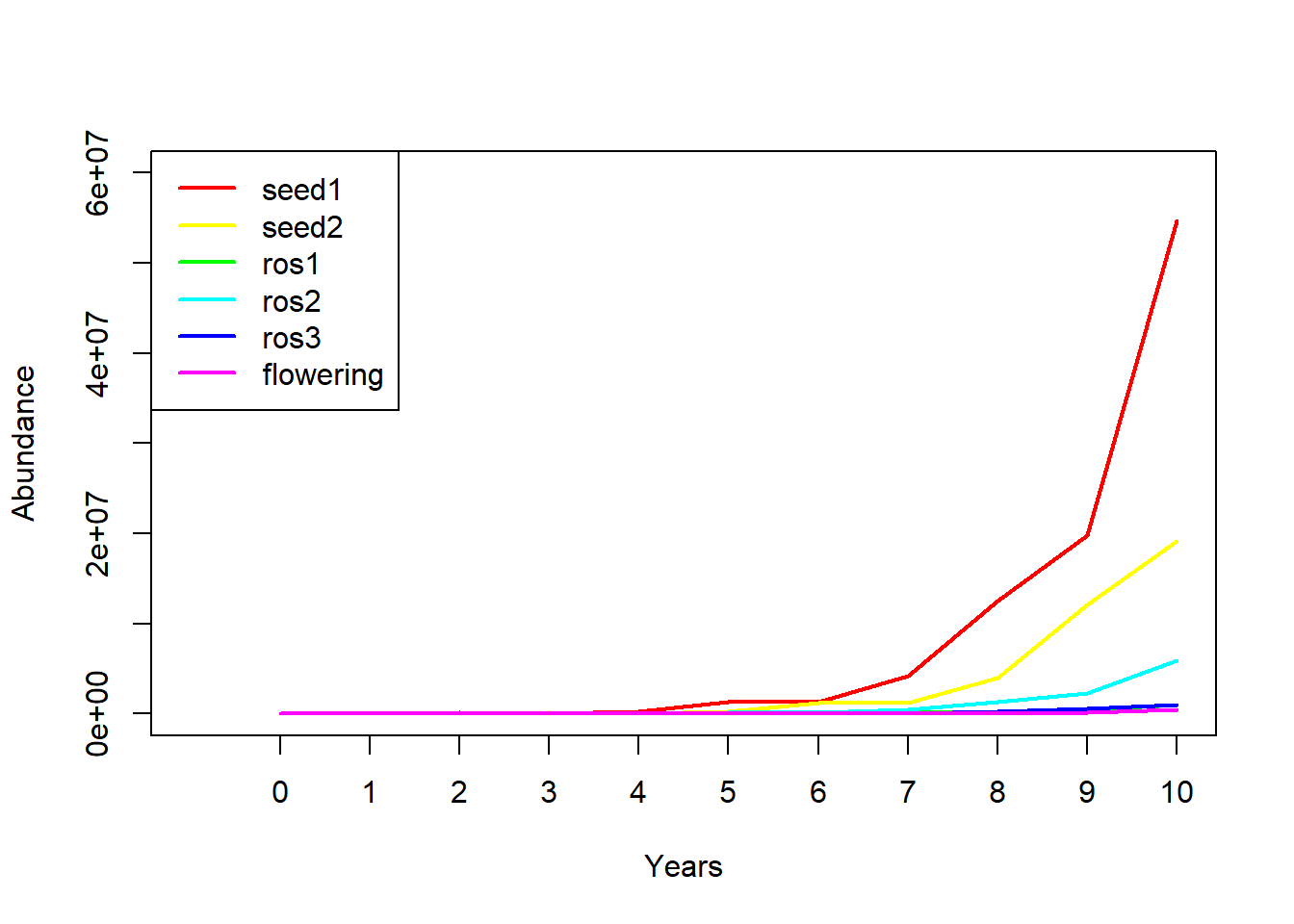So projection is easy with matrices!

### Reason 3: Matrix algebra tricks!

There is a clear similarity between the finite population growth equation:

$$N_{t+1}=\lambda \cdot N_t$$,

where $$N$$ is abundance (as always), $$t$$ is time, often in years but could be any time units, and $$\lambda$$ is the multipicative growth rate over the time period $$t \rightarrow t+1$$

… and the matrix population growth equation:

$$\mathbf{N}_{t+1} = \mathbf{A} \cdot \mathbf{N}_{t}$$,

where $$\mathbf{N}$$ is a vector of abundances (abundance for all stages), and $$\mathbf{A}$$ is the transition matrix, which we have seen before.

Q: Can you see the similarity between these two equations?

Both equations describe simple exponential growth or decline!

Q: Can you see the difference between these two equations?

Note that $$N$$ in the first equation is a scalar – that is, it is just a naked number with no additional components.

WHEREAS,

$$\mathbf{N}$$ in the second equation is an age-structured vector: a set of abundances structured by age or stage class.

Similarly, the finite population growth rate, $$\lambda$$ is a scalar,

WHEREAS,

$$\mathbf{A}$$ is a matrix (the transition matrix)

#### What about those tricks you promised??

Okay one of the tricks is this:

In one step, you can compute $$\lambda$$ from $$\mathbf{A}$$!!

All you need to do is obtain the first, or dominant, eigenvalue of $$\mathbf{A}$$! This number is the finite rate of growth, $$\lambda$$, for an age or stage-structured population.

Recall that when a population is at stable age distribution, it grows in a discrete exponential growth pattern- this rate of exponential growth can be described by a single parameter – Lambda!

Let’s do this in R!

What is the growth rate $$\lambda$$ for the teasel population. If you recall, it looked like it was growing, so it should be above 1…

###########
# Use the transition matrix to compute Lambda, or the finite rate of population growth!

Lambda <- as.numeric(round(eigen(teasel_matrix)$values,2)) Lambda ##  2.32 Or we could use the handy “popbio” package in R: library(popbio) # or... it's easier to use the 'popbio' library in R! ## Warning: package 'popbio' was built under R version 3.6.2 ## ## Attaching package: 'popbio' ## The following object is masked _by_ '.GlobalEnv': ## ## teasel lambda(teasel_matrix) ##  2.32188 You don’t have to understand the math here- but I do want you to understand how simple that was- just one line of code and we computed the annual rate of growth from the teasel transition matrix! Here’s another nifty trick: In one step, you can compute stable age distribution (S.A.D) from $$\mathbf{A}$$!! All you need to do is obtain the right-hand eigenvector of $$\mathbf{A}$$! This vector represents the relative abundances in each age class at the stable age distribution. Let’s do this in R! What is the stable age distribution for the teasel population. If you recall, the first seed stage looked like it dominated in the figure above. ########## # Compute stable age distribution from the transition matrix! SAD <- abs(as.numeric(round(eigen(teasel_matrix)$vectors[,1],3)))
SAD/sum(SAD)      # stable age distribution as a percentage of the total population
##  0.636615811 0.264909847 0.012482663 0.069348128 0.011789182 0.004854369

Or you can use the ‘popbio’ package in R:

library(popbio)    # ... and it's even easier if we use the 'popbio' package...
stable.stage(teasel_matrix)
##       Seed1       seed2        ros1        ros2        ros3   flowering
## 0.636901968 0.264978062 0.012174560 0.069281759 0.012076487 0.004587164

Q: Does a stage-structured population grow at the rate of $$\lambda$$ per time step if it is NOT at stable age distribution? [tophat]

To answer this question, you may find it helps to load an stage-structured model in InsightMaker like this one).

## Mechanics of matrix population models

Let’s take a look at a basic age-structured population – specifically the in-class example from the last lecture (this one.). In InsightMaker it looks something like this: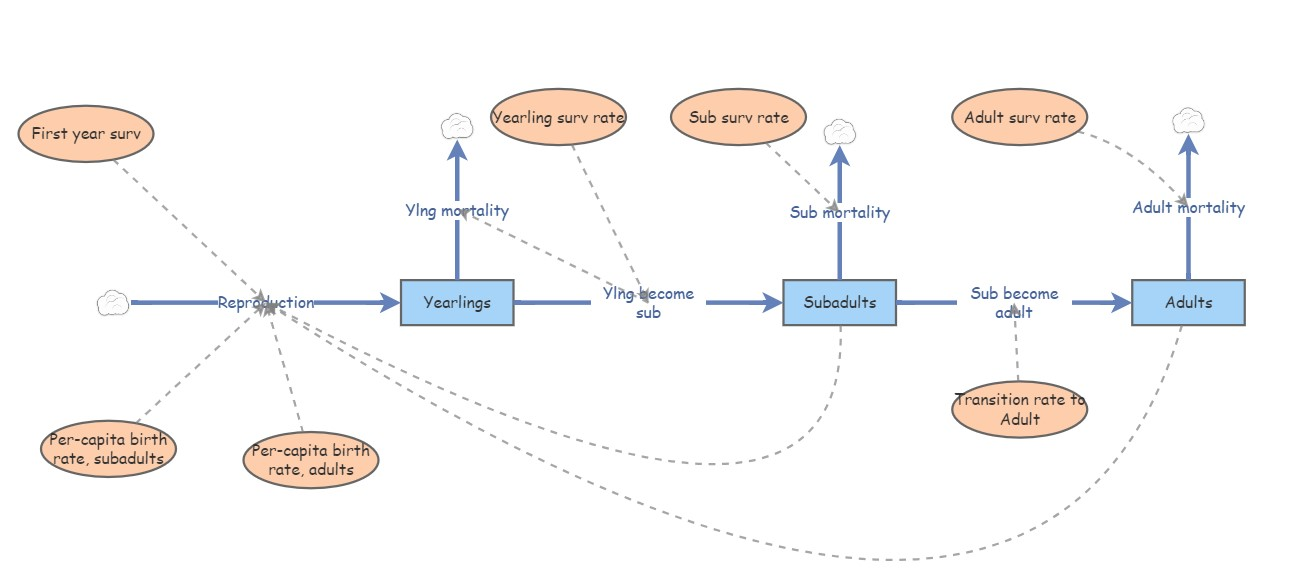Let’s convert the vital rates to a three-stage projection matrix. Projection matrices are square matrices where the number of rows and columns are equal to the number of life stages. In this case, that means three! Let’s make a blank matrix for now:

###################
# In class demo: convert an insightmaker model to a matrix projection model

###########
# First, we specify a blank transition matrix

TMat <- matrix(0,nrow=3,ncol=3)                    # create a blank matrix with 3 rows and 3 columns
rownames(TMat) <- stagenames
colnames(TMat) <- stagenames
TMat                                               # now we have an all-zero transition matrix.
##           Juveniles Subadults Adults
## Juveniles         0         0      0
## Adults            0         0      0

You can read the elements of a transition matrix as follows:

“The per-capita production of (row name) by (col name) is (value of element)

Now we can start filling in this matrix. Let’s begin with the top left element of the matrix. This represents the per-capita production of Juveniles (col) by Juveniles (row). What is the value of this element?

Let’s update our transition matrix:

#####
# fill in the top left element of the matrix

TMat[1,1] <- 0
TMat
##           Juveniles Subadults Adults
## Juveniles         0         0      0
## Adults            0         0      0

How about the second row, first column. This represents the per-capita production of Subadults (row) by previous-year Juveniles (col). That is, the transition rate from juvenile to subadult. The value from our model is 0.3.

Let’s update our transition matrix:

#####
# update the second row, first column

TMat[2,1] <- 0.3
TMat
##           Juveniles Subadults Adults
## Juveniles       0.0         0      0
## Adults          0.0         0      0

If we keep going, we get the following matrix. See if you can understand what this matrix is saying about the transitions from and two the three life stages.

#####
# and keep filling it in...

TMat[,1] <- c(0,0.3,0)          # fill in the entire first column of the transition matrix
TMat[,2] <- c(0,0.4,0.1)        # fill in the entire second column of the transition matrix
TMat[,3] <- c(4,0,0.85)         # fill in the entire third column of the transition matrix
TMat
##           Juveniles Subadults Adults
## Juveniles       0.0       0.0   4.00
## Adults          0.0       0.1   0.85

Now we can run a 40-year projection and compare it with the InsightMaker model. It had better look the same!!

First we must specify the initial abundances in each stage:

######
# specify initial abundance vector

InitAbund <- c(40,0,0)
names(InitAbund) <- colnames(TMat)
InitAbund
## Juveniles Subadults    Adults
##        40         0         0

So we are starting with only Juveniles…

#######
# Run the model for 40 years (using for loop)

nYears <- 40
allYears <- matrix(0,nrow=nrow(TMat),ncol=nYears+1)
rownames(allYears) <- rownames(TMat)
colnames(allYears) <- seq(0,nYears)
allYears[,1] <- InitAbund

for(t in 2:(nYears+1)){
allYears[,t] <-  TMat %*% allYears[,t-1]
}

allYears
##            0  1   2    3     4       5        6        7        8        9
## Juveniles 40  0 0.0 4.80 6.000 5.86800 5.871000 6.063630 6.287558 6.502333
## Subadults  0 12 4.8 1.92 2.208 2.68320 2.833680 2.894772 2.976998 3.077066
## Adults     0  0 1.2 1.50 1.467 1.46775 1.515907 1.571889 1.625583 1.679445
##                 10       11       12       13       14       15       16
## Juveniles 6.717782 6.940941 7.172411 7.411727 7.658952 7.914392 8.178354
## Subadults 3.181526 3.287945 3.397460 3.510707 3.627801 3.748806 3.873840
## Adults    1.735235 1.793103 1.852932 1.914738 1.978598 2.044588 2.112781
##                 17       18       19       20       21       22        23
## Juveniles 8.451123 8.732991 9.024259 9.325242 9.636263 9.957658 10.289772
## Subadults 4.003042 4.136554 4.274519 4.417085 4.564407 4.716641  4.873954
## Adults    2.183248 2.256065 2.331310 2.409066 2.489414 2.572443  2.658241
##                  24        25        26        27        28        29
## Juveniles 10.632962 10.987600 11.354065 11.732753 12.124071 12.528440
## Subadults  5.036513  5.204494  5.378077  5.557450  5.742806  5.934344
## Adults     2.746900  2.838516  2.933188  3.031018  3.132110  3.236574
##                  30        31        32        33        34        35
## Juveniles 12.946297 13.378090 13.824284 14.285360 14.761814 15.254160
## Subadults  6.132270  6.336797  6.548146  6.766543  6.992225  7.225434
## Adults     3.344522  3.456071  3.571340  3.690454  3.813540  3.940731
##                  36        37        38        39        40
## Juveniles 15.762926 16.288661 16.831930 17.393319 17.973432
## Subadults  7.466422  7.715446  7.972777  8.238690  8.513472
## Adults     4.072165  4.207983  4.348330  4.493358  4.643223

Now let’s plot it out!

#####
# and plot out the results!

plot(1,1,pch="",ylim=c(0,50),xlim=c(0,nYears+1),xlab="Years",ylab="Abundance",xaxt="n")
cols <- rainbow(3)
for(s in 1:3){
points(allYears[s,],col=cols[s],type="l",lwd=2)
}
axis(1,at=seq(1,nYears+1),labels = seq(0,nYears))
legend("topleft",col=cols,lwd=rep(2,3),legend=rownames(allYears))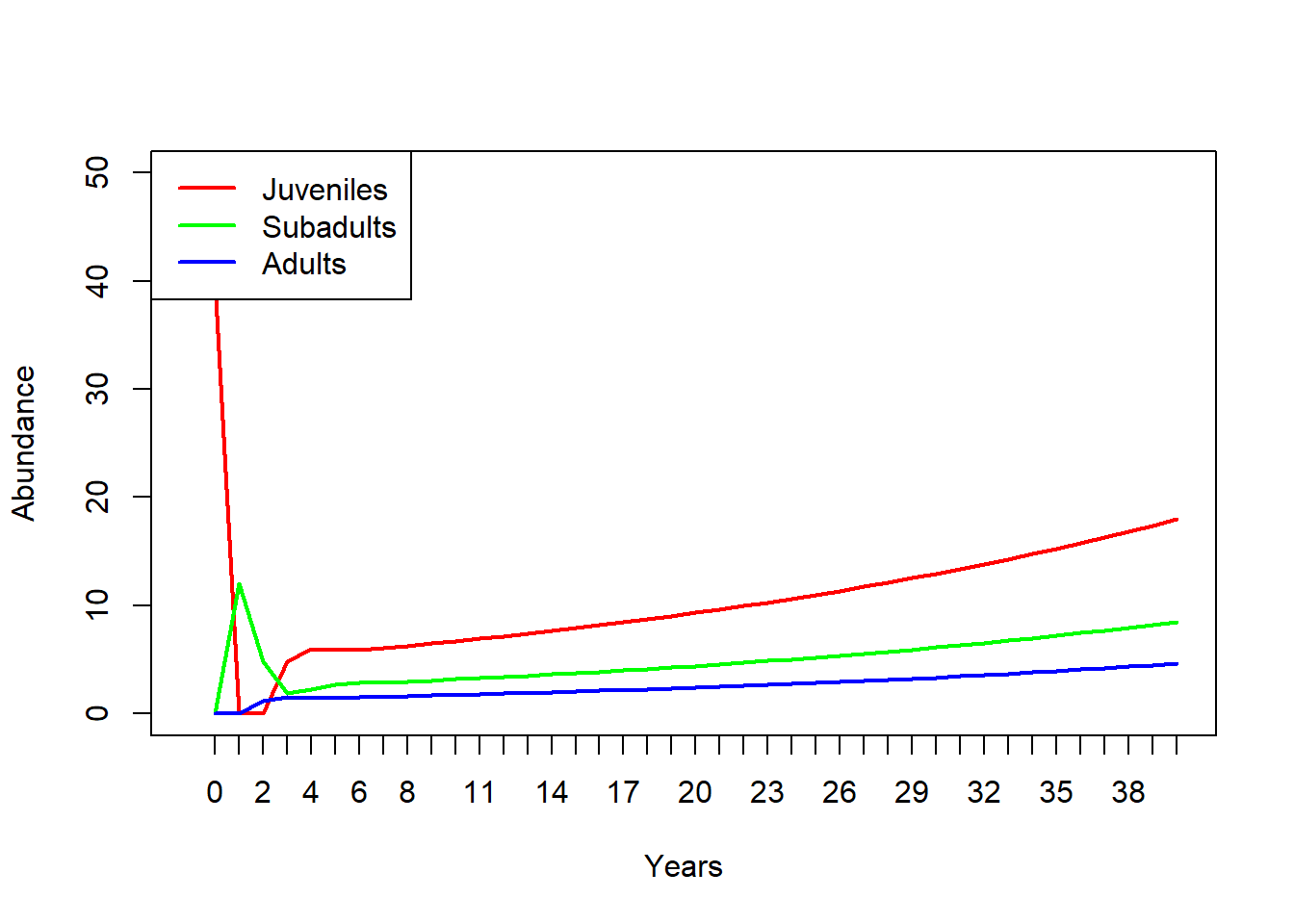Does this look the same as the InsightMaker results?

## Limitations of matrix population models

Matrix population models are great, but they have some limitations too.

In some ways, while introducing a new level of realism in our models – age-structure – we have been ignoring another type of realism that we introduced in earlier lectures- density-dependence!

Which vital rates are density-dependent? All? Some? It depends? Are the data available?

How do you incorporate density-dependence into a matrix population model?

How do you incorporate predator-prey dynamics into a matrix population model? [cue brain explosion]

Whatever you can do with a matrix population model, you can also do in InsightMaker (or other programming platform) (but it might be complicated and messy)

The reverse is NOT true: you can not always convert InsightMaker models to matrix population models (IM is a programming language, so is much more flexible!)

### Review of matrix multiplication

(on the whiteboard)

### In-class exercise: matrix projection models

Translate the following paragraph into a matrix population model. Remember a matrix population model has two components- an initial abundance vector and a transition matrix.

NOTE: this is also a question in Lab 4!We assumed that the Red-tailed hawk life history could be described in terms of three major life stages: hatchling (first year of life), juvenile (largely individuals in their second year of life), and adult (generally the third year of life and beyond). Adults are the primary reproductive stage, and produce an average of 3 new hatchlings each year. Juveniles are expected to produce only 1 new hatchling each year. We assumed that adults experienced an average of 18% mortality each year. Juvenile mortality was set at 30% per year. Approximately 5% of juveniles remain in the juvenile phase each year, and all other survivors transition to the adult stage. Finally, hatchlings had a 20% chance of surviving and transitioning to become juveniles. We initialized the population with 1000 hatchlings, 150 juveniles, and 5 adults.

Q: What does the transition matrix look like? [tophat]

Q: What does the initial stage abundance vector look like?

Q: Is this population at a stable stage-distribution?

Q: What is the growth rate of this population?

For more on matrix population models, the bible of this subject is this book by Hal Caswell.

And finally, check this out- this is a database of thousands of stage matrices for plants and animals around the world: